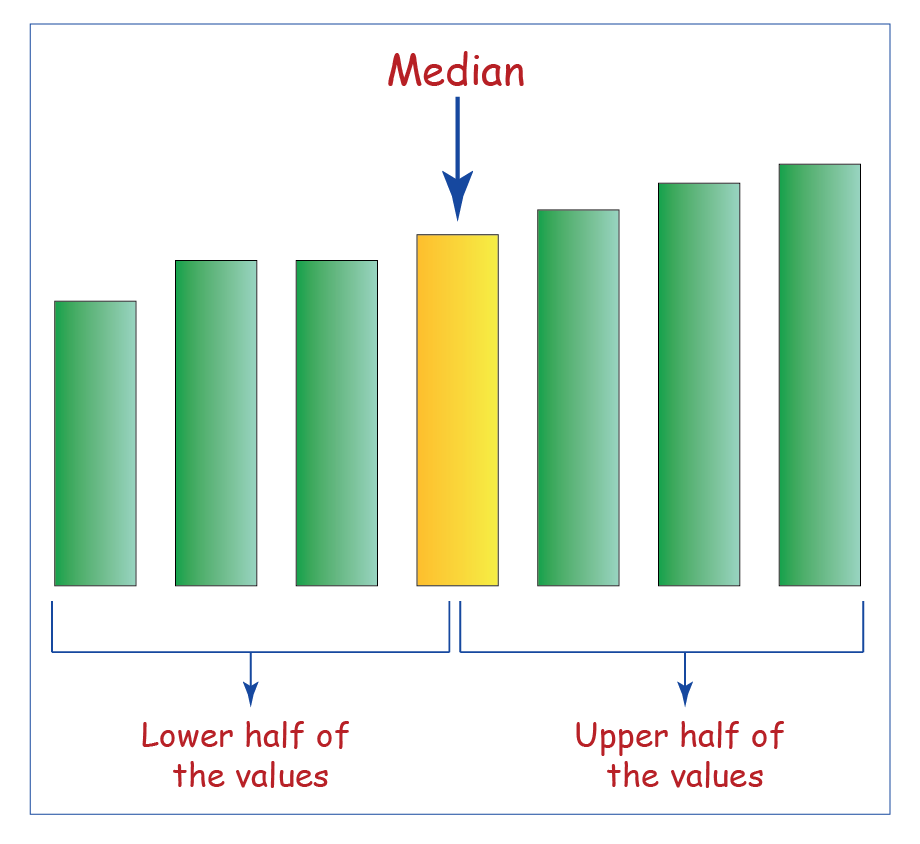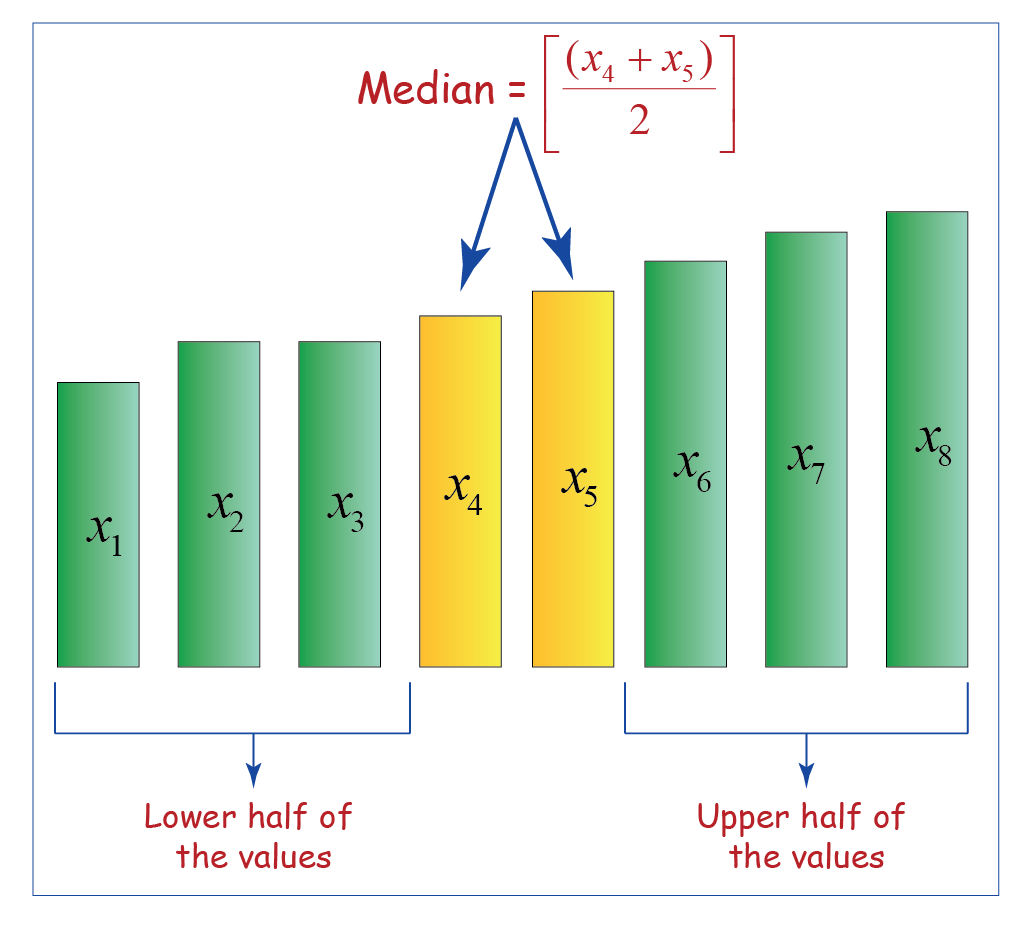# Median

Median

## Introduction

When describing a set of data, the central position of the data set is identified. This is known as the central measure of tendency. The three most common measures of central tendency are mean, median and mode.

Know more about the measures of central tendency.

Learn about the Mean and the Mode.

## Median

The median is the middle value of a set of numbers arranged in increasing order.

For finding this measure of central tendency, it is necessary to write the components of the group in increasing order.

### Case 1:

Let’s consider a data set with $$n$$ observations.

If $$n$$ is odd, then

\begin{align} \boxed{ \;\;\; \text{Median} = \frac{(n +1)}{2}^\text{th} \text{term} \;\;\;} \end{align}### Case 2:

Let’s consider a data set with $$n$$ observations.

If $$n$$ is even, then

\begin{align} \boxed{ \;\;\; \text{Median} = \frac{\left(\frac{n }{2}\right)^\text{th} \text{ term} + \left(\frac{n }{2}+1\right)^\text{th} \text{ term}}{2} \;\;\;} \end{align}## Solved Examples

### Example 1

The height (in centimeters) of the members of a school football team have been listed below.

{$$142,\;140,\;130,\;150,\;160,\;135,\;158,\;132$$}

Find the median of the above set.

### Solution:

Step 1:

Arrange the data items in ascending order.

Original set:

{$$142,\;140,\;130,\;150,\;160,\;135,\;158,\;132$$}

Ordered Set:

{$$130,\;132,\;135,\;140,\;142,\;150,\;158,\;160$$}

Step 2:

Count the number of observations.

Number of observations, $$n = 8$$

If number of observations is even, then we will use the following formula:

\begin{align} \text{Median} = \frac{\left(\frac{n }{2}\right)^\text{th} \text{ term} + \left(\frac{n }{2}+1\right)^\text{th} \text{ term}}{2} \end{align}

Step 3:

Calculate the median using the formula.

\begin{align} \text{Median} &= \frac{\left(\frac{n }{2}\right)^\text{th} \text{ term} + \left(\frac{n }{2}+1\right)^\text{th} \text{ term}}{2}\\ &=\frac{\left(\frac{8 }{2}\right)^\text{th} \text{ term} + \left(\frac{8 }{2}+1\right)^\text{th} \text{ term}}{2}\\ &=\frac{4^\text{th} \text{ term} + 5^\text{th} \text{ term}}{2} \\ &=\frac{140 + 142}{2}\\ &=141\end{align}

\begin{align} \boxed {\;\;\;\text{Median} = 141\;\;\;} \end{align}

### Example 2

The age of the members of a weekend poker team have been listed below.

{$$42, 40, 50, 60, 35, 58, 32$$}

Find the median of the above set.

### Solution:

Step 1:

Arrange the data items in ascending order.

Original set:

{$$42, 40, 50, 60, 35, 58, 32$$}

Ordered Set:

{$$32, 35, 40, 42, 50, 58, 60$$}

Step 2:

Count the number of observations.

Number of observations $$n = 7$$

If number of observations is odd, then we will use the following formula:

\begin{align}\text{Median}={\frac{(n +1)}{2}}^\text{th}\text{term}\end{align}

Step 3:

Calculate the median using the formula.

\begin{align}\text{Median}&={\frac{(n +1)}{2}}^\text{th}\text{term}\\ &={\frac{(7 +1)}{2}}^\text{th}\text{term}\\ &=4^\text{th}\text{term}\\ &=42 \end{align}

\begin{align}\boxed{\;\;\;\text{Median}=42\;\;\;} \end{align}

## Practice Questions

### Question 1

The table below shows the runs scored by the Indian batsmen during a one-day match. Calculate the median of the data.

 Cricketer Runs Scored Virat Kohli $$56$$ Irfan Pathan $$15$$ Virendra Sehwag $$51$$ M.S. Dhoni $$25$$ Sachin Tendulkar $$78$$

Hint: The number of observations is odd.

### Question 2

The table below shows the amount of money donated for an old age home by different grades of Model School. Calculate the median of the data.

 Grade Amount of money donated (in Rs) Grade 1 $$1000$$ Grade 2 $$750$$ Grade 3 $$900$$ Grade 4 $$1500$$ Grade 5 $$2000$$ Grade 6 $$1700$$ Grade 7 $$1750$$ Grade 8 $$2500$$ Grade 9 $$1800$$ Grade 10 $$3000$$

Hint: The number of observations is even.

More Important Topics
Numbers
Algebra
Geometry
Measurement
Money
Data
Trigonometry
Calculus
More Important Topics
Numbers
Algebra
Geometry
Measurement
Money
Data
Trigonometry
Calculus
Learn from the best math teachers and top your exams

• Live one on one classroom and doubt clearing
• Practice worksheets in and after class for conceptual clarity
• Personalized curriculum to keep up with school